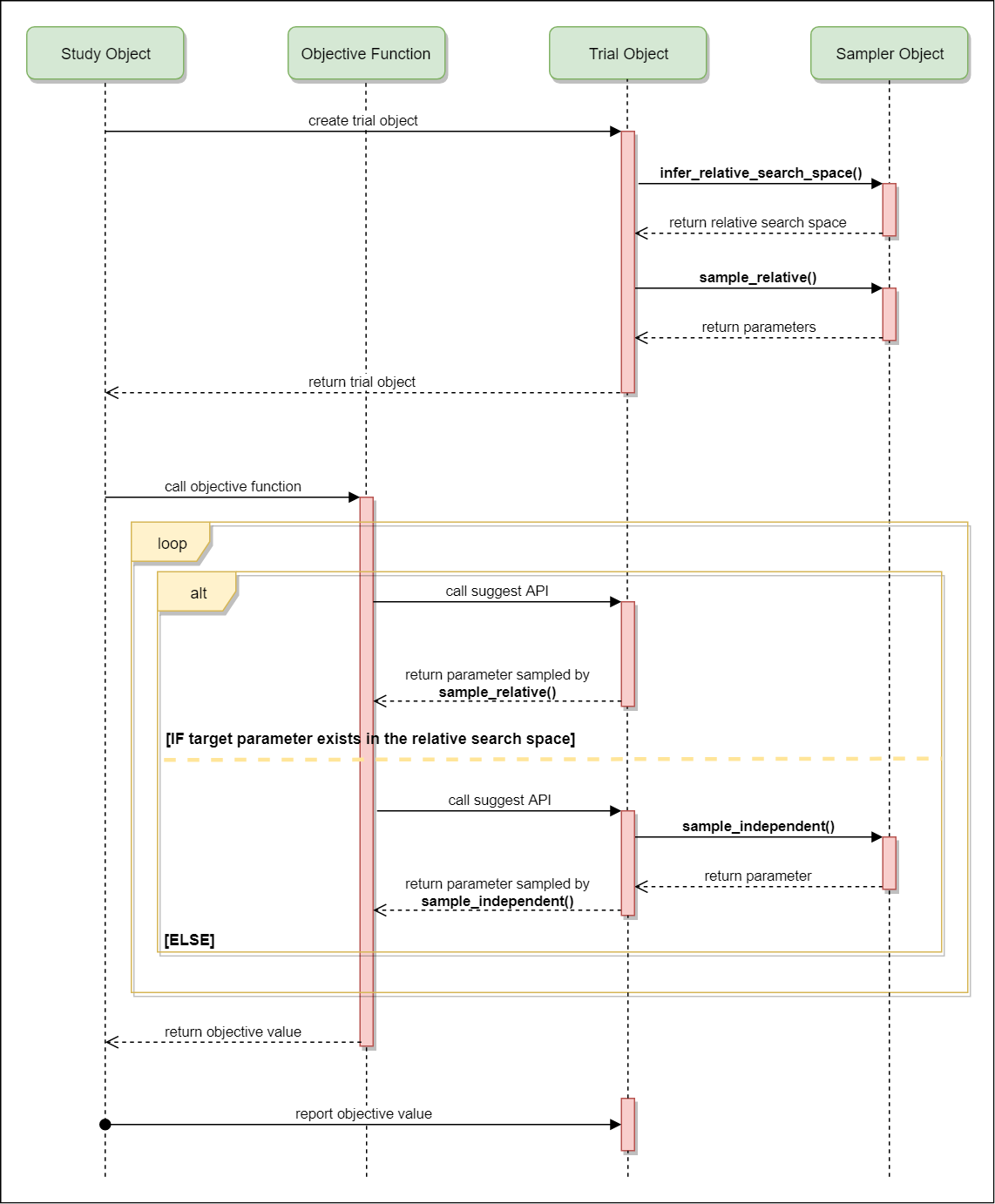# Samplers¶

class optuna.samplers.BaseSampler[source]

Base class for samplers.

Optuna combines two types of sampling strategies, which are called relative sampling and independent sampling.

The relative sampling determines values of multiple parameters simultaneously so that sampling algorithms can use relationship between parameters (e.g., correlation). Target parameters of the relative sampling are described in a relative search space, which is determined by infer_relative_search_space().

The independent sampling determines a value of a single parameter without considering any relationship between parameters. Target parameters of the independent sampling are the parameters not described in the relative search space.

More specifically, parameters are sampled by the following procedure. At the beginning of a trial, infer_relative_search_space() is called to determine the relative search space for the trial. Then, sample_relative() is invoked to sample parameters from the relative search space. During the execution of the objective function, sample_independent() is used to sample parameters that don’t belong to the relative search space.

The following figure depicts the lifetime of a trial and how the above three methods are called in the trial.infer_relative_search_space(study, trial)[source]

Infer the search space that will be used by relative sampling in the target trial.

This method is called right before sample_relative() method, and the search space returned by this method is pass to it. The parameters not contained in the search space will be sampled by using sample_independent() method.

Parameters: study – Target study object. trial – Target trial object. A dictionary containing the parameter names and parameter’s distributions.
sample_independent(study, trial, param_name, param_distribution)[source]

Sample a parameter for a given distribution.

This method is called only for the parameters not contained in the search space returned by sample_relative() method. This method is suitable for sampling algorithms that do not use relationship between parameters such as random sampling and TPE.

Parameters: study – Target study object. trial – Target trial object. param_name – Name of the sampled parameter. param_distribution – Distribution object that specifies a prior and/or scale of the sampling algorithm. A parameter value.
sample_relative(study, trial, search_space)[source]

Sample parameters in a given search space.

This method is called once at the beginning of each trial, i.e., right before the evaluation of the objective function. This method is suitable for sampling algorithms that use relationship between parameters such as Gaussian Process and CMA-ES.

Parameters: study – Target study object. trial – Target trial object. search_space – The search space returned by infer_relative_search_space(). A dictionary containing the parameter names and the values.
class optuna.samplers.RandomSampler(seed=None)[source]

Sampler using random sampling.

This sampler is based on independent sampling. See also BaseSampler for more details of ‘independent sampling’.

Example

>>> study = optuna.create_study(sampler=RandomSampler())
>>> study.optimize(objective, direction='minimize')

Args:
seed: Seed for random number generator.
class optuna.samplers.TPESampler(consider_prior=True, prior_weight=1.0, consider_magic_clip=True, consider_endpoints=False, n_startup_trials=10, n_ei_candidates=24, gamma=<function default_gamma>, weights=<function default_weights>, seed=None)[source]

Sampler using TPE (Tree-structured Parzen Estimator) algorithm.

This sampler is based on independent sampling. See also BaseSampler for more details of ‘independent sampling’.

On each trial, for each parameter, TPE fits one Gaussian Mixture Model (GMM) l(x) to the set of parameter values associated with the best objective values, and another GMM g(x) to the remaining parameter values. It chooses the parameter value x that maximizes the ratio l(x)/g(x).

For further information about TPE algorithm, please refer to the following papers:

Example

import optuna
from optuna.samplers import TPESampler

def objective(trial):
x = trial.suggest_uniform('x', -10, 10)
return x**2

study = optuna.create_study(sampler=TPESampler())
study.optimize(objective, n_trials=100)

static hyperopt_parameters()[source]

Return the the default parameters of hyperopt (v0.1.2).

TPESampler can be instantiated with the parameters returned by this method.

Example

Create a TPESampler instance with the default parameters of hyperopt.

import optuna
from optuna.samplers import TPESampler

def objective(trial):
x = trial.suggest_uniform('x', -10, 10)
return x**2

sampler = TPESampler(**TPESampler.hyperopt_parameters())
study = optuna.create_study(sampler=sampler)
study.optimize(objective, n_trials=100)

Returns: A dictionary containing the default parameters of hyperopt.
optuna.samplers.intersection_search_space(study)[source]

Return the intersection search space of the BaseStudy.

Intersection search space contains the intersection of parameter distributions that have been suggested in the completed trials of the study so far. If there are multiple parameters that have the same name but different distributions, neither is included in the resulting search space (i.e., the parameters with dynamic value ranges are excluded).

Returns: A dictionary containing the parameter names and parameter’s distributions.
optuna.samplers.product_search_space(study)[source]

Return the product search space of the BaseStudy.

Deprecated since version 0.14.0: Please use intersection_search_space() instead.